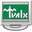# College Scientific Calculator 361.0.1.8

Developed by - Windows - setup file CollegeScientificCalculator36.exe (1.32 MB) - Shareware

MD5: 660ddb2e59843fba23b7f426954f8e10

## Developer Description

"College Scientific Calculator 36"

A handy, fast, reliable, precise tool if you need to perform complex mathematical calculations. Scientific Calculator Precision 36 is programmed in C#. All calculations are done in proprietary data type. The calculator handles mathematical formulas of any length and complexity. Calculation history can be stored into text file or printed. There are ten variables or constants available for storing often used numbers. Precision of calculations is 36 digits. Trigonometric, hyperbolic, inverse and combinatorial functions. Special numbers NaN, Uncertainty, and Infinity. The calculator follows classical approach when uncertainty of f(x) calculation is estimated by formula max|(derivative(f))|*|x*uncertainty(x)|, where maximum of function derivative is considered on interval [x-uncertainty(x),|x+uncertainty(x)], and uncertainty(x)=|x|*10^(-precision).

What's new in this version: version checker is added

### Antivirus information

Download3K has downloaded and tested College Scientific Calculator 36 on 10 Jan 2015 with some of Today's best antivirus engines and you can find below, for your convenience, the scan results:

Clean

Clean

Clean

Clean
User rating